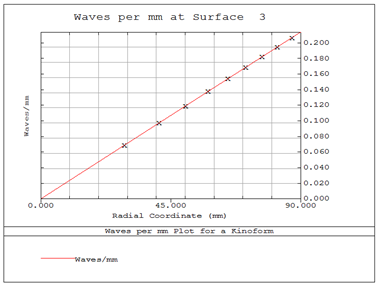## Calculating the Sag of a Diffractive Optical Element Using a ZPL Macro in OpticStudio

Category: Product NewsThis blog post explains how to calculate the sag of a diffractive optical element using a ZPL macro in OpticStudio.

We will describe the use of a macro to calculates the sag and phase data of a rotationally symmetric kinoform (Binary 2 surface) lens surface. These surface types are commonly used in infrared imaging systems, and also have uses in visible applications, such as intraocular lenses. User inputs for the macro include the surface number and radial step size. Outputs include the zone number, zone radius, inner radius sag, outer radius sag, and step height for the diffractive optic. Profile frequency (in waves/mm) (which can be used as manufacturing difficulty merit) is also calculated.

Equations and definitions

The general form of the sag of a diffractive surface is calculated as follows1: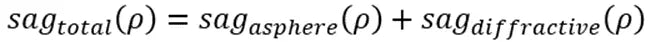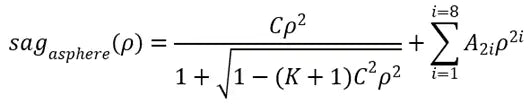where:

=  conic constant

A2,4,6,8…  =  aspheric coefficients

λ  =  wavelength

N  =  refraction index of lens

C2,4,6,8…  =  phase coefficients

Step height is given as: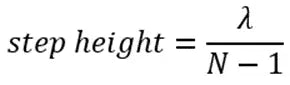Phase profile of a diffractive surface in radians is given as: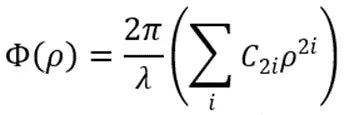OpticStudio uses the normalized form of phase coefficients. The conversion of normalized form is given below, with R representing the normalized radius:The typical diffractive profile is as follows:Inputs

Once activated, the macro takes the surface number and radius iteration step size for sag calculations from the user, as shown below: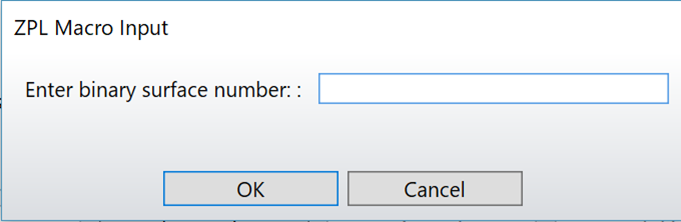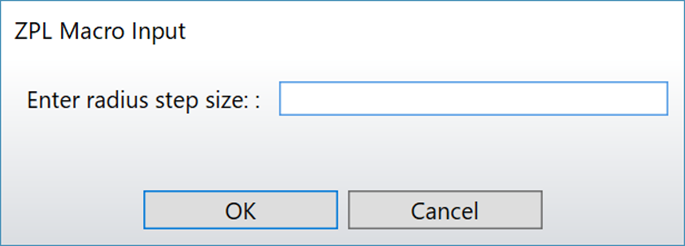Outputs

The macro outputs zone number, zone radius, inner radius sag, outer radius sag, step height, and profile frequency (waves/mm) as shown below: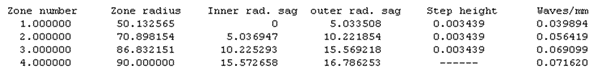This is followed by the calculated data for each radius incremented by the step size, as shown below:The macro plots the phase, integer part of phase, and phase modulo 1 wave as shown below: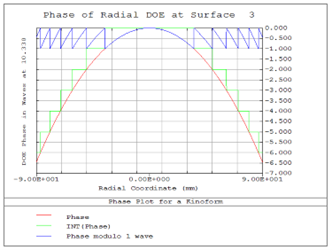Finally, profile frequency in waves/mm is plotted and frequencies corresponding to zone radius are crossed as shown below: